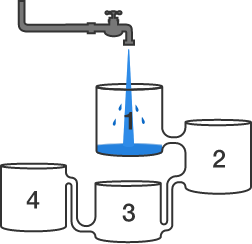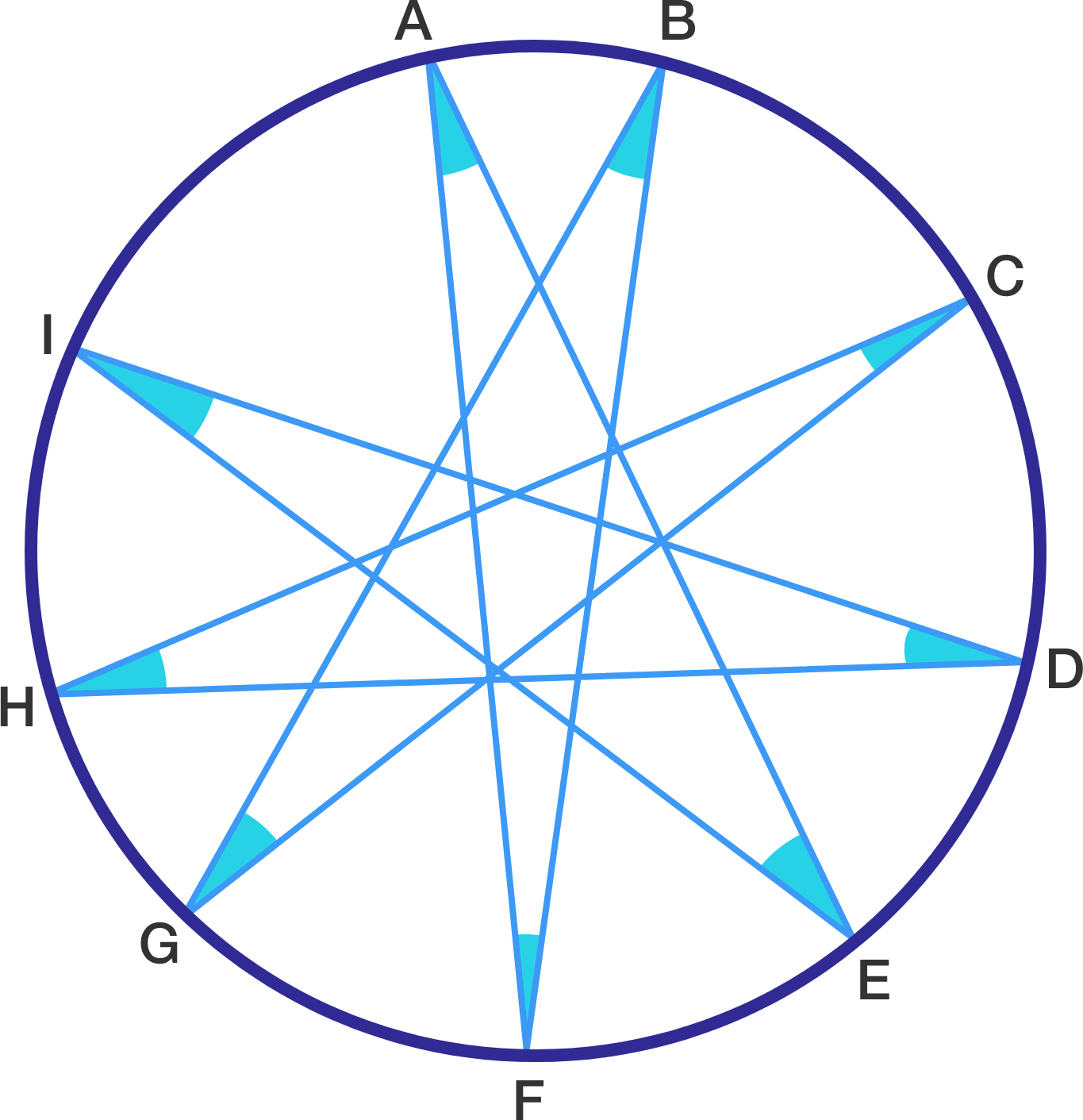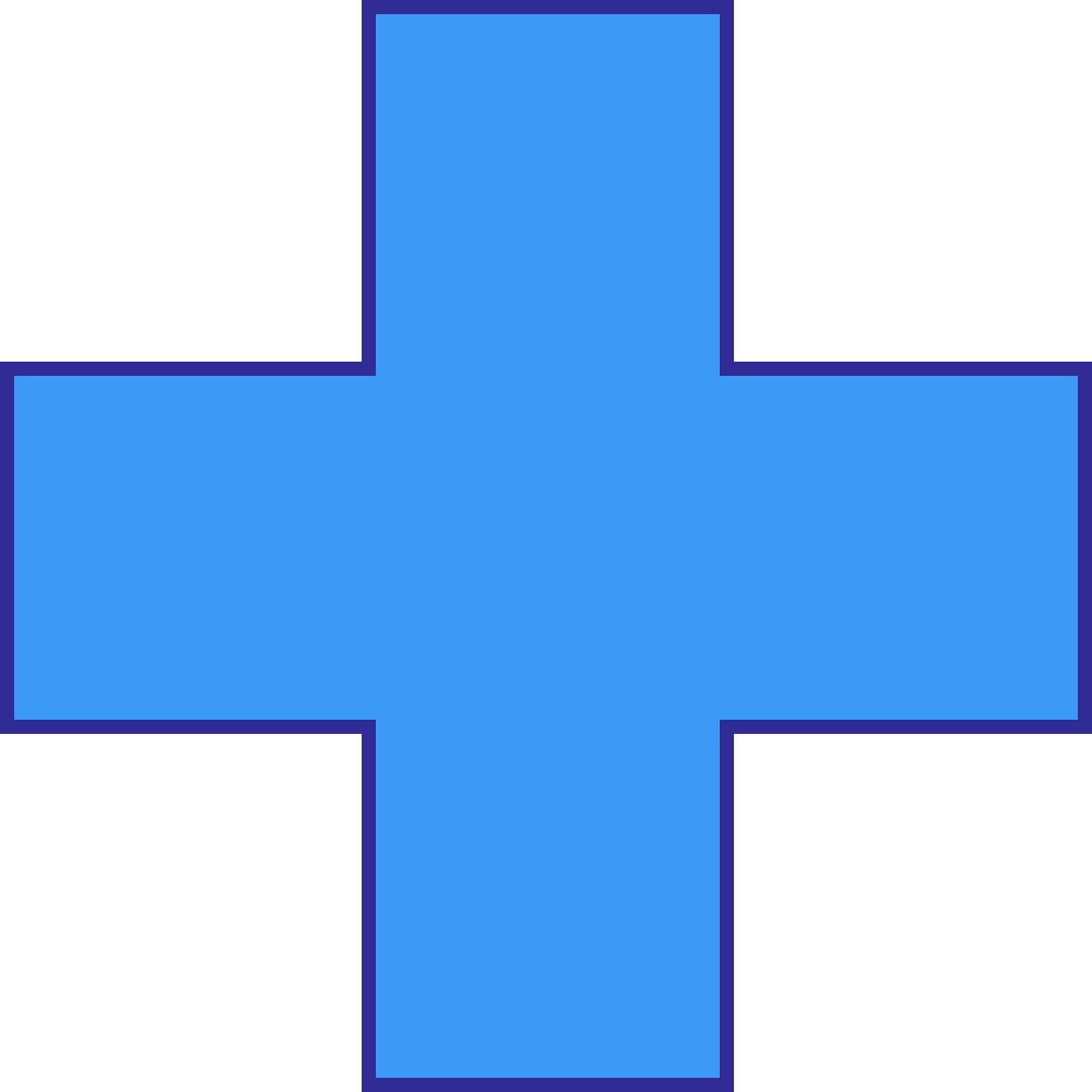# Problems of the Week

Contribute a problem

# 2017-04-24 BasicFour open containers of equal volume are connected as shown.

If the capacity of the pipes is greater than the flowrate from the tap, which container will fill first?

$\begin{array} { l l l } & A & B \\ + & C & D \\ \hline & E & F \\ \end{array}$

In the above cryptogram, all the letters represent distinct digits.

What is the minimum possible value of the 2-digit integer $\overline{EF}?$

Note: In cryptogram problems, 0 is not allowed to be a leading (first) digit, since we would just not write anything in its place.If points $A, B, C, D, E, F, G, H, I$ all lie on a circle, as shown above, find $\angle A+\angle B+\angle C+\angle D+\angle E+\angle F+\angle G+\angle H+\angle I$ in degrees.

$\begin{array} { ccccccc } 1234 & 1243 & 1324 & 1342 & 1423 & 1432 \\ \vdots & & & & & \vdots \\ 4123 & 4132 & 4213 & 4231 & 4312 & 4321 \\ \end{array}$

The 24 distinct 4-digit numbers above have been listed out by arranging 1, 2, 3, and 4 in different orders. What is the sum of these 24 numbers?


Hint: You do not need to list out all 24 numbers to find the sum.

###### From: Derek Niederman's Math Puzzles for the Clever MindBy using 2 straight lines, what is the most number of regions that we can separate the cross into?

Note: Using 1 straight line, it is possible to separate the cross into 3 regions.

×